# How to use the IF function in Google SheetsOf all the functions that Google Sheets provides, the IF function is one of the most useful. Just like the IF formula in Excel, you can use it to perform logical tests on your spreadsheet data. This allows you to carry out different actions and provide different answers if the test result is TRUE or FALSE.

You can use a group of IF statements together (nested IF) and you can also use IF function in combination with other logical functions such as AND and OR.

IF returns a value through an if-then-else logical construct. Firstly, it evaluates the cell or range of cells to see if they meet the criteria in the logical expression. If the result is TRUE, the formula returns a value, performs a calculation, or executes another function. If the result is FALSE, the function acts differently, returning a different value or carrying out a different calculation or formula.

Essentially, you are telling the function: If the cell meets the criteria, do X, otherwise do Y. You can set your criteria and your resulting actions to be whatever you want.

See how this process works in the flow chart below: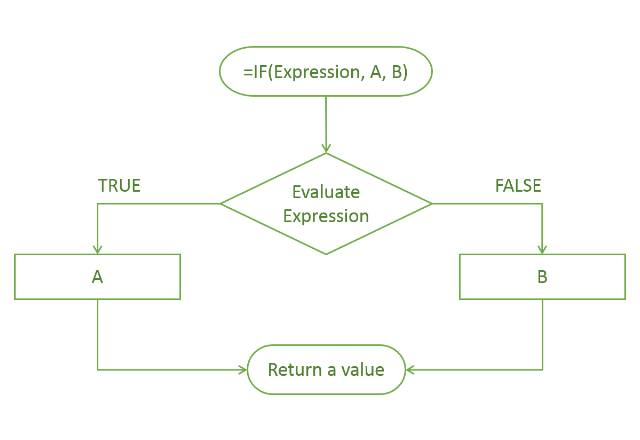### Syntax

IF(logical_expression, value_if_true, value_if_false)

• logical_expression – an expression or reference to a cell containing an expression that has a logical value such as TRUE or FALSE.
• value_if_true – the value that the function returns if the logical_expression is TRUE. This can be a number, text, or even another function that returns a value. You can also embed another IF statement in here: this is known as a nested IF.
• value_if_false – this is the value that the IF function returns if the logical_expression is FALSE. Similar to the value_if_true, this can be a number, text, or another formula that returns a value. It can also be a nested IF. Please note that this is an optional input and if you leave it out you will get a blank value in return.

### How to use Google Sheets IF function

#### Example 1: Regular IF statements

The easiest way to understand the IF function is to see it in action.

Take a look at the following sample data (columns A through E). In column F you can see some common variations of the function.

You will notice that I have experimented with Boolean values, dates, numbers, and also text. Boolean values have only two possible variables: true or false.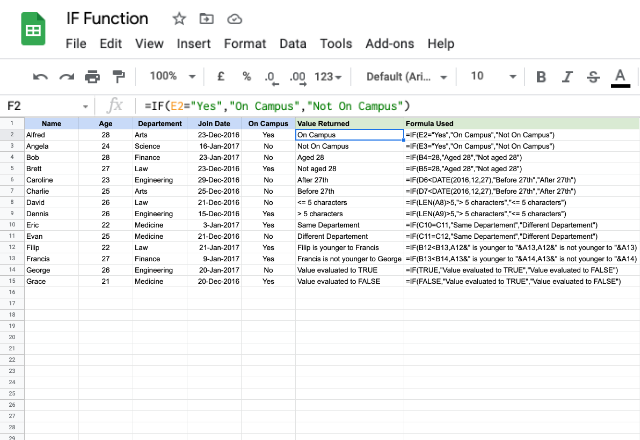There are two rows for each example, to show you the result of the function depending on whether the value is true or false. To get you started, I’ll explain the first two rows.

The equation used in the first two rows is =if(E2=”Yes”,”On Campus”,”Not On Campus”).

To put this in simple terms, I am telling the function “If the value in cell E2 is “Yes”, then the output should be “On Campus”, otherwise (if the value in cell E2 is not Yes) the output should be “Not On Campus”.

By taking a look at all of the examples, you will get an idea of the versatility of the IF function. You can use it with just about any other function: you can see the DATE function in rows 6 & 7, and the LEN (length of a text string) function in rows 8 & 9.

It can also use a variety of logical tests to evaluate whether an expression is TRUE or FALSE. The logical tests that you can use are listed here: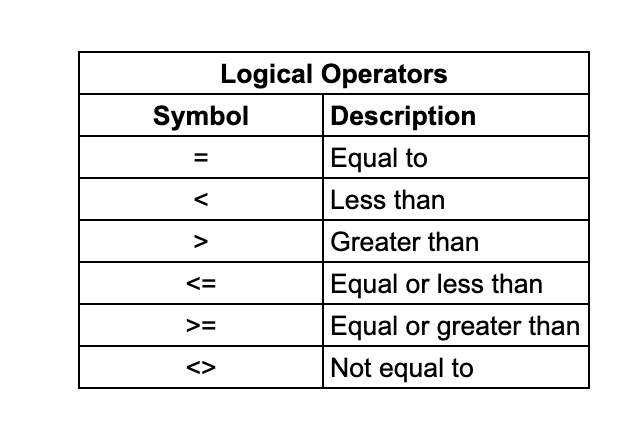#### Example 2: IF statements with a calculation as the result

You can also use an IF statement to return a mathematical calculation.

In the following example, I’m evaluating the final grade of a group of students, based on whether or not they receive bonus marks. If they do, their final grade will increase by the percentage indicated, and if they don’t, their final grade will stay the same.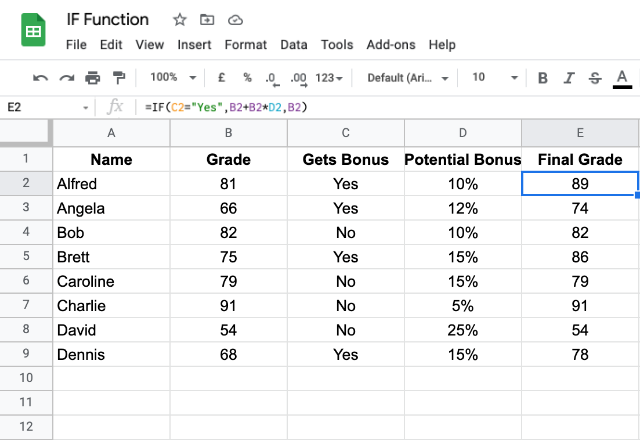The equation I use to do this is =IF(C2=”Yes”, B2+B2*D2, B2).

Notice that in this case, the value_if_true is actually a calculation that the function must perform.

### Example 2: Nested IF statements

Quite often, you may want to use a series of IF statements in a logical sequence. This is known as a nested IF statement. You can see this in the following diagram: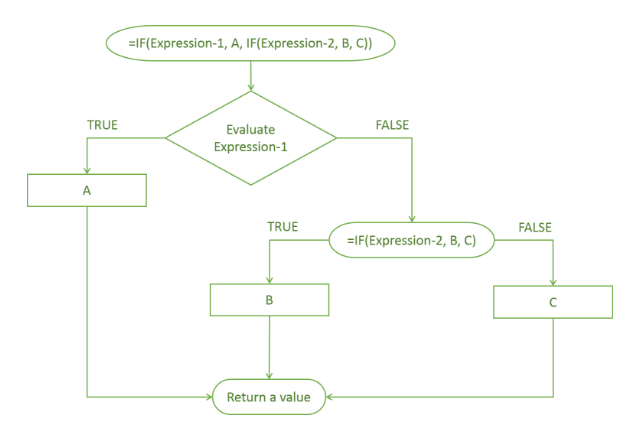As you can see in this flow chart, I have nested an IF within the value_if_false. You can also nest an IF within the value_if_true.

In my example, if the IF test for Expression-1 is FALSE, the function goes to the next test: the nested IF function. Accordingly, it returns either B or C based on whether the test for Expression-2 is TRUE or FALSE.

This diagram shows a single nested IF statement. But you can also try multi-level nesting. This means listing multiple IF functions in a hierarchical fashion.

Take a look at the example below: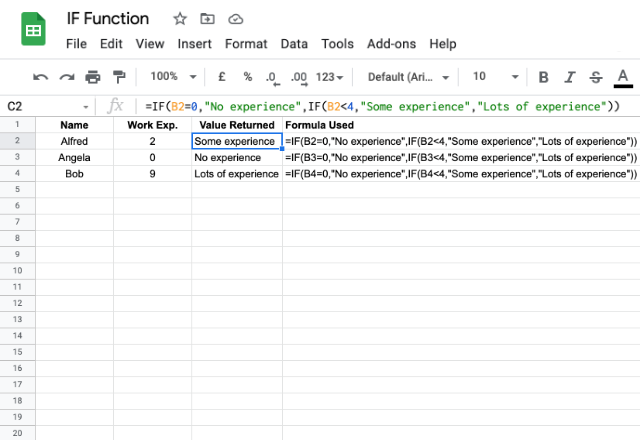In this example, I am using an IF statement to evaluate how much experience each worker has.

I do this with the equation =if(B2=0,”No experience”,if(B2<4,”Some experience”,”Lots of experience”)).

Again, to put this in simple terms, I am telling the function: If the value in cell B2 is 0, then output “No experience”, otherwise (if B2 is not 0), if the value in B2 is less than 4, output “Some experience”, otherwise (if B2 is not less than 4) output “Lots of experience”.

#### Example 3: IF functions with AND & OR functions

The AND and OR functions in Google Sheets are logical functions similar to the IF function, and they can be used in combination with the IF function. These two expressions are fairly self-explanatory: use an AND function when you want a cell to meet multiple criteria, and an OR function when you want a cell to meet at least one out of multiple criteria.

#### IF with AND function

Here’s an example of an IF function that also uses an AND function.

The equation here is =IF(AND(B2>19,B2<24),”Early 20’s”,IF(AND(B2>23,B2<27),”Mid 20’s”,IF(AND(B2>26,B2<30),”Late 20’s”,”Not in their 20’s”))).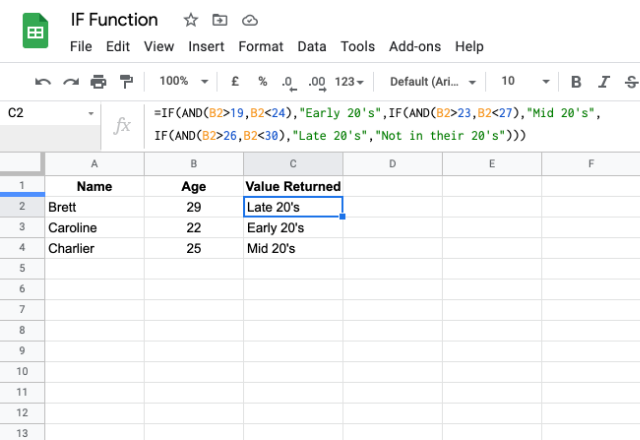I have used the AND function because I want the age cell to be tested against two criteria in my logical test: a minimum value and a maximum value. By doing so, I am able to separate the ages into segments that I’m calling “Early 20s”, “Mid 20s”, and “Late 20s”.

Notice that I’ve also included “Not in their 20s” as the value_if_false at the end of the equation. This will make sure that any data that does not fit the criteria I’ve set (any ages that are not in the 20s) will still have an output that makes sense.

#### IF with OR function

I’ll now show an example using the OR function within an IF statement. Here, I’m evaluating the overall experience of workers, where anyone who is either above the age of 25 or has more than 4 years of work experience, is deemed to be experienced.

I accomplish this by typing =if(OR(B2>25,C2>4),”Experienced”,”Not experienced”).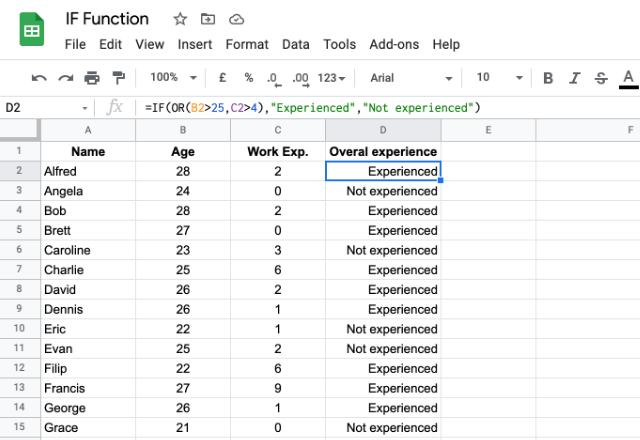Note the difference between this example and the previous one. Here, I want the value to be TRUE (i.e. the worker to be deemed “experienced”) if either one of the two criteria are met. This is why I use the OR function. If I wanted both criteria to be met for the value to be TRUE, I would use the AND function (like in the previous example).

#### IFS Function

IF function helps you apply logical functions to your spreadsheet data with simple TRUE or FALSE decision-making. Nested IFs can be used when you want to test data against multiple sets of criteria, but they can become very long and complex.

Luckily there’s an alternative: the IFS function in Google Sheets.

#### Did you like this post?

If you found this article useful, share it with your friends, colleagues, and fellow spreadsheet users via the social media buttons on the left!

Editor’s note: This is a revised version of a previous post that has been updated for accuracy and comprehensiveness.